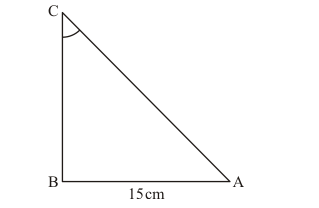# Given 15 cot A = 8, find sin A and sec A.

Question:

Given 15 cot A = 8, find sin A and sec A.

Solution:

Given: $15 \cot A=8$

To Find: $\sin A, \sec A$

Since $15 \cot A=8$

By taking 15 on R.H.S

We get,

$\cot A=\frac{8}{15} \ldots \ldots .(1)$

By definition,

$\cot A=\frac{1}{\tan A}$

Hence,

$\cot A=\frac{1}{\frac{\text { Perpendicular side opposite to } \angle \mathrm{A}}{\text { Base side adjacent to } \angle \mathrm{A}}}$

$\cot A=\frac{\text { Base side adjacent to } \angle \mathrm{A}}{\text { Perpendicular side opposite to } \angle \mathrm{A}}$.....(2)

Comparing equation (1) and (2)

We get,

Base side adjacent to $\angle \mathrm{A}=8$

Perpendicular side opposite to $\angle \mathrm{A}=15$

$\triangle A B C$ can be drawn as shown below using above informationHypotenuse side AC is unknown.

Therefore, we find side $\mathrm{AC}$ of $\triangle A B C$ by Pythagoras theorem.

So, by applying Pythagoras theorem to $\triangle A B C$

We get,

$A C^{2}=A B^{2}+B C^{2}$

Substituting values of sides from the above figure

$A C^{2}=8^{2}+15^{2}$

$A C^{2}=64+225$

$A C^{2}=289$

$A C=\sqrt{289}$

$A C=17$

Therefore, Hypotenuse = 17

Now by definition,

$\sin A=\frac{\text { Perpendicular side opposite to } \angle \mathrm{A}}{\text { Hypotenuse }}$

Therefore, $\sin A=\frac{B C}{A C}$

Substituting values of sides from the above figure

$\sin A=\frac{15}{17}$

By definition,

$\sec A=\frac{1}{\cos A}$

Hence,

$\sec A=\frac{1}{\frac{\text { Base side adjacent to } \angle \mathrm{A}}{\text { Hypotenuse }}}$

$\sec A=\frac{\text { Hypotenuse }}{\text { Base side adjacent to } \angle \mathrm{A}}$.

Substituting values of sides from the above figure

$\sec A=\frac{17}{8}$

Answer: $\sin A=\frac{15}{17}$ and $\sec A=\frac{17}{8}$